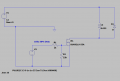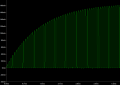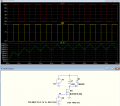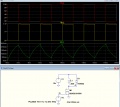# Inductor / Coil / PWM door lock understand issues.

#### Gorden

Joined Oct 30, 2019
27
Hi,

I have returned to an old issue I am struggling with – my door lock solenoid project again.

I have created a really simple LTSpice circuit driving my solenoid at 1Khz, 50% duty. The solenoid I measured with an inductance meter, 35mH and 25R series resistance, this has been entered into the inductor parameters.

I did not add any parallel resistance, because to be honest I don’t understand this.

I am measuring the current through the 0.1R resistor.

If I drive the MOSFET at 100%. The current through the 0.1R is as expected, 480mA. When I go the PWM drive, I expect to see a square wave of 0-480mA 1Khz 50%.

But, that’s not what I see, I am seeing an averaged version of this, as in the attached “Coil2” image. This shows 180mA.

So, I am trying to understand the physics of exactly what is happening here – I have assume the inductance of the coil, along with the various resistances means that the rate of change within the coil is creating this effect.

From a previous post, I see that the time constant will be

t=L/R, so t=0.035/25= 0.0014s =1.4mS

The questions I am trying to answer/understand is:-

What does this time constant actually mean – is this the slope I see when the signal is first applied, up to 63%, is this the current building in coil due to the inductance/resistance and then the average current will be set by the frequency applied / the rate of change?

What will the actual current through the coil be, 480mA pulses, or as seen across the resistor?

Does this mean that the average current seen by the power supply will be even lower than my expected 50%, because if we are starting with 180mA and not 480mA, 50% could be as low as 90mA. (which is approximately what I see if I add a CLC filter onto this circuit).

If I actually build this and measure the voltage across the resistor, I see square waves of approx. 25mV, so 250mA which I guess could be close as the simulation does not take account of the power supply etc.

It’s the fundamental process I need to understand, if anyone can help.#### BobTPH

Joined Jun 5, 2013
6,523
If I drive the MOSFET at 100%. The current through the 0.1R is as expected, 480mA. When I go the PWM drive, I expect to see a square wave of 0-480mA 1Khz 50%.
Then you di not understand how an inductor behaves.

Let’s look at the 100% case first. You say the current is 480mA as expected. But that is only true after infinite time has passed. When you first turn the circuit on, the current is zero. It then increases at a rate based on the inductance. The time constant refers to a simplification of the behavior. It says that it will reach 63% of the final current after one time constant. After that, each time constant will see it increase by 63% of the remaining difference between the the starting current and the final current.

Now look at the PWM case. At 1KHz and 50% duty cycle, it will be in for only 0.5ms, considerably less than one time constant. So the current will not reach even 50%.

#### LowQCab

Joined Nov 6, 2012
3,077
Door-Locks are usually not designed to have their Power Pulse-Width-Modulated.

There are 2 usual methods of handling a PWMed-Inductor.
The first would be a "Freewheeling-Diode",
and the second would be a "Snubber-Circuit".

But any method of controlling the Power to a Door-Lock is
likely to cause the Door-Lock to fail to actuate, or release, reliably.

What problem are You trying to solve by controlling the Power to the Door-Lock ?
.
.
.

#### Gorden

Joined Oct 30, 2019
27
BobTPH,

You are correct - i did not understand how the inductor is behaving.

Thank you for the explanation - i need to play with the simulation timings to see this.

But, if i decrease the frequency to 250Hz 50%, now the coil will be on for 2ms - what would i expect to see?

Actaully doing this (i can only go 300hz to 10khz), measuring the current across the resistor does increase the current by a small amount.

If the current does have time to the full charge, why is it only 50% and not pulses of 100%, 50% of the time - i do realize it will not be, but i really want to understand the detail of why.

LowQcab,

The door lock mechanism and coil is being used to hold a automatic shutter in place, it is powered for long periods, and this method reduces the current and heating. It does work fine - it's just my understanding of how it works thats failing!

Many thanks.

#### BobTPH

Joined Jun 5, 2013
6,523
But, if i decrease the frequency to 250Hz 50%, now the coil will be on for 2ms - what would i expect to see?
The current would rise to something greater than 63% of 480mA in each period.

Last edited:

#### LowQCab

Joined Nov 6, 2012
3,077
You can think of an Inductor as a mechanical-device, sort-of like a Flywheel.
It stores Power in a Magnetic-Field,
( instead of a static-potential-Charge, like a Capacitor ).

Inductors and Capacitors can complement each other if they are sized correctly.

The goal of a properly designed "L-C" Filter is to "average" the Power going through it.
Ideally, with a pulsating Input, ( PWM ),
it should output a Voltage that is a "Flat-Line" DC-Voltage-Level that
is the average of the On-Time, and Off-Time of the Pulses.

Since your Circuit is not properly set up in an ideal manner as an "L-C-Filter",
You are only seeing a partial, weird manifestation of this Filtering effect.

You didn't state what your Power-Supply consists of.
If it's AC, the quick and dirty solution is to just power the Solenoid with AC through a Diode,
this will cut the overall Power going to the Coil in half.

The Door-Latch-Solenoid should have a "Free-Wheeling-Diode" placed across the Coil in any Case,
( except when Powering the Coil with an AC-Voltage ),
whether it's PWM-Regulated, or just a Diode,
after which, You will have to pay particular attention to having the correct Supply-Polarity.
If the Polarity is not correct, the Diode will be a direct-Short-Circuit across the Power-Supply,
and could cause some "Magic-Blue-Smoke" to leak out of something.

If 50% Power created by a Diode, is not enough for reliable operation,
then install a 1000uf Capacitor across the Solenoid to increase the average Power.

Do some research on how a "Buck-Regulator" works,
this will demonstrate how an Inductor works with a Capacitor, and a Freewheeling-Diode
to create "mostly smooth" DC-Voltage from a Pulsating DC-Voltage.
.
.
.

#### BobaMosfet

Joined Jul 1, 2009
2,073
Hi,

I have returned to an old issue I am struggling with – my door lock solenoid project again.

I have created a really simple LTSpice circuit driving my solenoid at 1Khz, 50% duty. The solenoid I measured with an inductance meter, 35mH and 25R series resistance, this has been entered into the inductor parameters.

I did not add any parallel resistance, because to be honest I don’t understand this.

I am measuring the current through the 0.1R resistor.

If I drive the MOSFET at 100%. The current through the 0.1R is as expected, 480mA. When I go the PWM drive, I expect to see a square wave of 0-480mA 1Khz 50%.

But, that’s not what I see, I am seeing an averaged version of this, as in the attached “Coil2” image. This shows 180mA.

So, I am trying to understand the physics of exactly what is happening here – I have assume the inductance of the coil, along with the various resistances means that the rate of change within the coil is creating this effect.

From a previous post, I see that the time constant will be

t=L/R, so t=0.035/25= 0.0014s =1.4mS

The questions I am trying to answer/understand is:-

What does this time constant actually mean – is this the slope I see when the signal is first applied, up to 63%, is this the current building in coil due to the inductance/resistance and then the average current will be set by the frequency applied / the rate of change?

What will the actual current through the coil be, 480mA pulses, or as seen across the resistor?

Does this mean that the average current seen by the power supply will be even lower than my expected 50%, because if we are starting with 180mA and not 480mA, 50% could be as low as 90mA. (which is approximately what I see if I add a CLC filter onto this circuit).

If I actually build this and measure the voltage across the resistor, I see square waves of approx. 25mV, so 250mA which I guess could be close as the simulation does not take account of the power supply etc.

It’s the fundamental process I need to understand, if anyone can help.

View attachment 287739View attachment 287740
This will help

Title: Understanding Basic Electronics, 1st Ed.
Publisher: The American Radio Relay League
ISBN: 0-87259-398-3

#### michael8

Joined Jan 11, 2015
339
When an inductor has a changing current it generates a voltage
opposing the current change. An equation is:
V = L * di/dt
Where V is the voltage across the inductor, L is the inductance,
and di/dt is the rate of change in the current.

So when you connect your 12 volt source to your 35mH and 25 ohm inductor
the first current which flows is zero. Since the current is zero the
voltage drop in the resistances is zero and the inductance sees the full
source voltage.

The current may start at zero but it's increasing at the rate of:

di/dt = V/L -> 12 volts / .035 H -> 324 amps/second (or 342 uA/uS).

Now at each instant, as the curent increases the voltage drop in
the circuit resistance increases descreasing the voltage the
inductor sees -- thus the rate of current increase will drop.
Long term this will give you the L/R time constant effect.

Your 50% 1KHz square wave isn't long term, it's only high for
500 uS so the most current which can flow will be:

342uA * 500 -> 171 mA

When your source voltage turns off (open circuit) the peak current will
(for an instant) continue at 171 mA. The voltage across the inductor
will "fly" up to whatever voltage is needed to maintain that current.
The current will then ramp down. Since the current is decreasing
the di/dt term is negative and the resulting inductor voltage will
be negative. If you have a diode across the inductor with the proper
polarity it will pass this current at the diode forward voltage,
perhaps 1 volt. So the down ranp will start:

di/dt = V/L -> 1 volt / .035 H -> 28 amps/second (or 2.8 uA/uSec).

Since this is slower than the ramp up it will take longer and likely
won't get done by the time the 2nd half of the 1KHz square wave
ends (and the inductor goes back to a current ramp up).

Hope that helps...

#### crutschow

Joined Mar 14, 2008
32,064
Here are two simulations of you circuit, one with a 1kHz square-wave and one with a 250Hz square-wave.
The ripple in the current through the inductor is caused by the time-constant of the inductor inductance and its series resistance.
The current increases at the time-constant rate when the transistor is on (current through transistor), and decreases by the time-constant rate when the transistor is off (current through diode).

Does that help with your understanding?#### LowQCab

Joined Nov 6, 2012
3,077
The best solution for limiting the long-term Temperature rise in the Door-Latch-Solenoid
is to use a DC-Current-Regulator-Circuit.

This can help to insure sharp/quick actuation while only supplying the
minimum required Current for holding the Solenoid open.

A Current-Regulator, "effectively", tends to make an Inductance act more like a Resistance,
this can make the Solenoid "appear to be more-linear",
rather than highly Reactive, in it's behavior.

What does your Power-Supply consist of ?, and the specifications of it ?
What Control-Circuitry do You already have in place, and, are You willing to change it ?
What is the DC-Resistance of the Solenoid-Coil ?, and any known specifications ?
What method(s) of control of the Solenoid would be desirable ?
Is this project "Fire-Safety" related in any way ?
.
.
.